Search IntMath
Close

450+ Math Lessons written by Math Professors and Teachers

5 Million+ Students Helped Each Year

1200+ Articles Written by Math Educators and Enthusiasts

Simplifying and Teaching Math for Over 23 Years

# Fractals

Your browser does not support HTML5 canvas.
Fractal animation courtesy of Rectangleworld

With computers, we can generate beautiful art from complex numbers. These designs, some of which you can see on this page, are called fractals.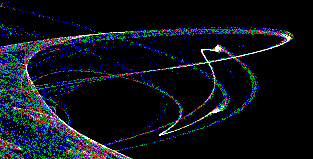Fractals are produced using an iteration process. This is where we start with a number and then feed it into a formula. We get a result and feed this result back into the formula, getting another result. And so on and so on...

Fractals start with a complex number. Each complex number produced gives a value for each pixel on the screen. The higher the number of iterations, the better the quality of the image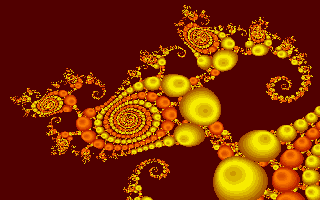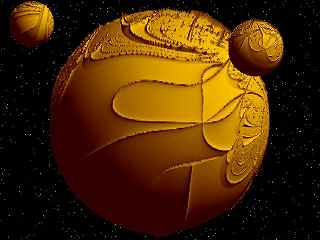Common fractals are based on the Julia Set and the Mandelbrot Set.

Continues below

## Julia Set

The Julia Set equation is:

Zn+1 = (Zn)2 + c

For the Julia Set, the value of c remains constant and the value of Zn changes.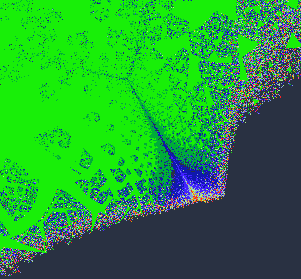### Example of a Julia Set:

If we start with the complex number Z1= 0.5 + 0.6j, and let c = 0.3 and then feed this into the formula above, we have:

Z2 = (0.5 + 0.6j)2 + 0.3 = 0.19 + 0.6j

We now take this new answer and feed it back in:

Z3 = (0.19 + 0.6j)2 + 0.3 = -0.0239 + 0.228j

Continuing, we find that

Z4 = 0.24858721 − 0.0108984j and

Z5 = 0.3616768258

− 0.005418405698j

## The Mandelbrot Set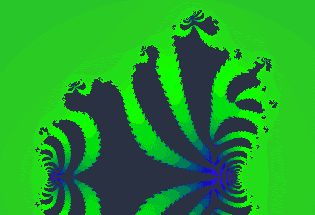The Mandelbrot Set (discovered accidentally by an IBM computer programmer of that name) is the same as the Julia Set, but the value of c is allowed to change.

## More fractals

### The Fractal FernThe "fractal fern" is generated completely by fractals. This is not a digital photograph - it is completely computer-generated.

### Autumn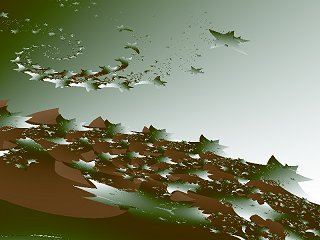Image source: Fractales

## Is There a Use for Any of This?

There certainly is.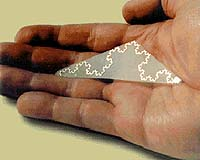A US company called Fractal Antenna Systems, Inc. makes antenna arrays that use fractal shapes to get superior performance characteristics, because they can be packed so close together.

This design ensures performance improvements in antennae used in wireless, microwave, RFID (Radio Frequency Identification) and telecommunications.

More details can be found at: http://www.spacedaily.com/news/antenna-02d.html